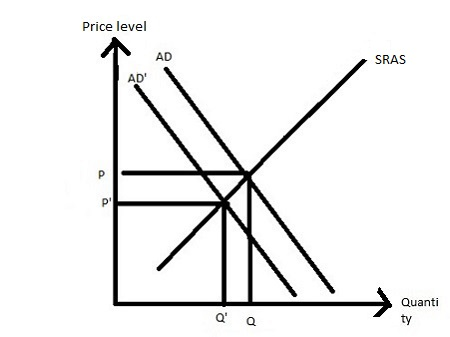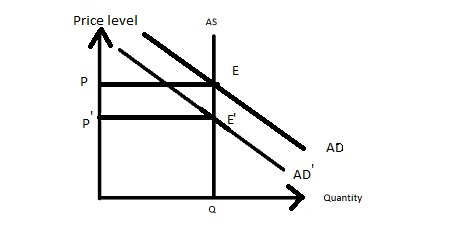# Consider the forces that led to the Great Depression. In the very short run, the effect of these...

## Question:

Consider the forces that led to the Great Depression. In the very short run, the effect of these forces would be what change in the price level and what change in real output?

none of the listed answers

a decrease; no change

an increase; a decrease

no change; a decrease

a decrease; a decrease

Consider again the Great Depression. The long run effect of the forces cited above would be what change in the price level and what change in real output?

a decrease; a decrease

none of the listed options

an increase; a decrease

no change; a decrease

a decrease; no change

## Aggregate supply:

In the short run, the aggregate supply curve is upward sloping such that as the price rises then the total output of all goods supplied rises and as the price falls then the total output of all good supplied also falls shows that the aggregate supply curve is upward sloping.

In the long run, the aggregate supply curve is vertical such that the economy is at its full-employment level of output where the real GDP is equal to potential GDP.

## Answer and Explanation:

In the short run, the depression occurs due to falling in the aggregate demand for goods and services with the same aggregate supply such that both price level and output decreases.So, a decrease; a decrease is correct.

In the long run, the aggregate supply curve is vertical such that the depression occurs due to falling the aggregate demanded for goods and services in such a way that the price level decreases and quantity remain the same.So, a decrease; no change is correct.

#### Learn more about this topic:Aggregate Supply and Aggregate Demand (AS-AD) Model

from Economics 102: Macroeconomics

Chapter 7 / Lesson 3
58K